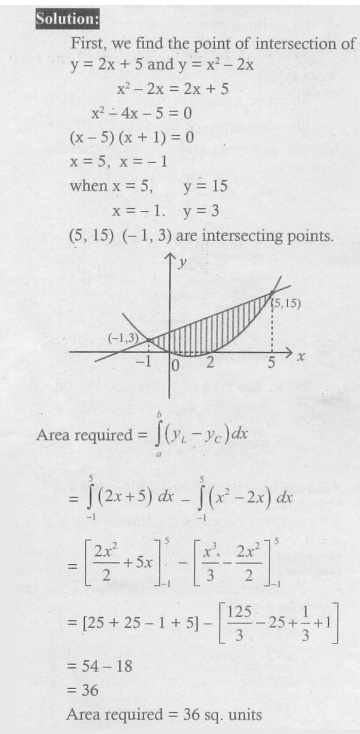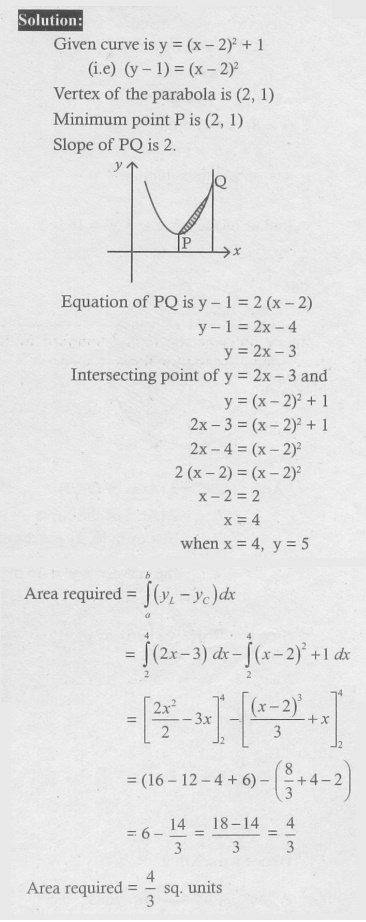Home | | Maths 12th Std | Exercise 9.8: Evaluation of a Bounded Plane Area by Integration

# Exercise 9.8: Evaluation of a Bounded Plane Area by Integration

Maths Book back answers and solution for Exercise questions - Mathematics : Applications of Integration: Evaluation of a Bounded Plane Area by Integration: Exercise Problem Questions with Answer, Solution

EXERCISE 9.8

1. Find the area of the region bounded by 3x ŌłÆ 2 y + 6 = 0 , x = ŌłÆ3 , x = 1 and x-axis.2. Find the area of the region bounded by 2x ŌłÆ y += 0 , y = ŌłÆ1, y = 3 and y-axis.3. Find the area of the region bounded by the curve 2 + x ŌłÆ x2 + y = 0 , x-axis, x = ŌłÆ3 and x = 3.4. Find the area of the region bounded by the line y = 2x + 5 and the parabola y = x2 ŌłÆ 2x .5. Find the area of the region bounded between the curves y = sin x and y = cos x and the lines x = 0 and x = ŽĆ .6. Find the area of the region bounded by y = tan x , y = cot x and the lines x = 0 , x = ŽĆ/2 , y = 0.7. Find the area of the region bounded by the parabola y 2 = x and the line y = x ŌłÆ 2 .8. Father of a family wishes to divide his square field bounded by x = 0 , x = 4 , y = 4 and = 0 along the curve y2 = 4and x2 = 4 y into three equal parts for his wife, daughter and son. Is it possible to divide? If so, find the area to be divided among them.9. The curve y = ( x ŌłÆ 2)2 +1 has a minimum point at P. A point Q on the curve is such that the slope of PQ is 2. Find the area bounded by the curve and the chord PQ.10. Find the area of the region common to the circle x2 + y2 = 16 and the parabola y2 = 6x .1. 7.5

2. 2

3. 15

4. 36

5. 2ŌłÜ2

6. logŌĆē2

7. 9/2

8. yes, 16/3

9. 4/3

10. 4/3 ( 4ŽĆ + ŌłÜ3)

Tags : Problem Questions with Answer, Solution , 12th Maths : UNIT 9 : Applications of Integration
Study Material, Lecturing Notes, Assignment, Reference, Wiki description explanation, brief detail
12th Maths : UNIT 9 : Applications of Integration : Exercise 9.8: Evaluation of a Bounded Plane Area by Integration | Problem Questions with Answer, Solution